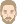## If it possible to output the transport coefficient used in the calculation

yuan
WUFI UserPosts: 11
Joined: Mon Jan 21, 2019 2:51 pm -1100

### If it possible to output the transport coefficient used in the calculation

Hi, thank you for your attention.
I was using WUFI Pro to calculate moisture transfer in ALC. I input the measured water content of ALC (6 points) and for the heat and moisture conductivity, I use the default value in WUFI. I know that WUFI use interpolation method to give Dww and water content in the calculation, but WUFI give a figure for the Dww and equilibrium relationship, and it seems like a linear relationship between two measured value. But I want to know the value used in the calculation. I can output the water content of a certain element at each time step. Is it possible to output the corresponding liquid transport coefficient used in the calculation?
I try to output the water content of two elements and the moisture flux on the surface to calculate the Dww, but the results are not reasonable. I can output the flux on the surface, but is it possible to output the flux between different elements?
Thank you!
Yuan

ThomasPosts: 301
Joined: Sun Jun 19, 2005 10:33 pm -1100

### Re: If it possible to output the transport coefficient used in the calculation

yuan wrote:
Tue Jan 21, 2020 8:12 pm -1100
I know that WUFI use interpolation method to give Dww and water content in the calculation, but WUFI give a figure for the Dww and equilibrium relationship, and it seems like a linear relationship between two measured value.
Hi Yuan,
WUFI uses linear interpolation to find water contents of the moisture storage function between the tabulated values.

It uses linear interpolation of the logarithms of the Dww to find values between the table entries. (Note that the interpolated segments of the Dww curve are shown as straight lines in the diagram which has a logarithmic y-axis.)
But I want to know the value used in the calculation. I can output the water content of a certain element at each time step. Is it possible to output the corresponding liquid transport coefficient used in the calculation?
The liquid transport coefficients can not be output. One reason is that the transport coefficients describe the moisture or heat transport between the grid elements, so they are not a property of any specific grid element.
I try to output the water content of two elements and the moisture flux on the surface to calculate the Dww, but the results are not reasonable. I can output the flux on the surface, but is it possible to output the flux between different elements?
You can divide an existing layer into two layers with identical material parameters in such a way that the new layer boundary passes between the two grid elements of interest. Then you can use the ASCII output to output the flux across this boundary.

Regards,
Thomas

yuan
WUFI UserPosts: 11
Joined: Mon Jan 21, 2019 2:51 pm -1100

### Re: If it possible to output the transport coefficient used in the calculation

Dear Thomas
Thank you so much, it helped me a lot. But I have another question: Is it means when I am calculating the moisture flux between two grid elements, the liquid transport coefficient is dependent on the average water content of the two grid elements?
Best regards
Yuan

Thomas